Next: Laplace's Equation in Cylindrical Up: Potential Theory Previous: Dirichlet Problem in Spherical

# Newmann Problem in Spherical Coordinates

According to Section 2.10, the solution to the Newmann problem, in which the charge density is specified within some volume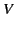, and the normal derivative of the potential given on the bounding surface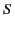, takes the form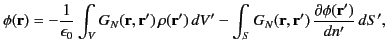(382)

where the Newmann Green's function is written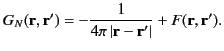(383)

Here,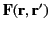is solution of Laplace's equation (i.e.,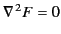) which is chosen so as to ensure that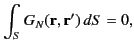(384)

and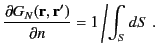(385)

The latter constraint holds when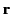(or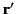) lies on. Note that we have chosen the arbitrary constant to which the potential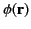is undetermined such that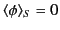. It again follows from Sections 3.4 and 3.5 that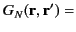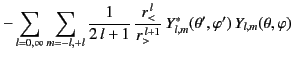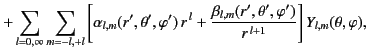(386)

where the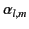and the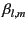are chosen in such a manner that the constraints (385) and (386) are satisfied.

As a specific example, suppose that the volumelies inside the spherical surface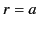. The physical constraint that the Green's function remain finite at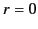implies that theare all zero. Applying the constraint (385) at, we get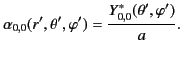(387)

Similarly, the constraint (386) leads to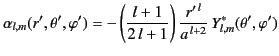(388)

for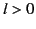. Hence, the unique Green's function for the problem becomes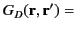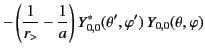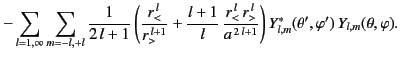(389)

Finally, expandingand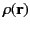in the forms (378) and (379), respectively, Equations (383) and (390) yield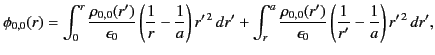(390)

and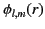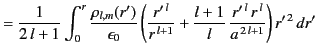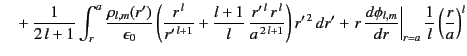(391)

for.Next: Laplace's Equation in Cylindrical Up: Potential Theory Previous: Dirichlet Problem in Spherical
Richard Fitzpatrick 2014-06-27